# First Order Non-homogeneous Differential Equation

An example of a first order linear non-homogeneous differential equation is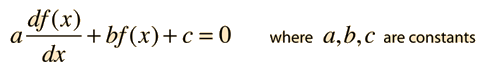Having a non-zero value for the constant c is what makes this equation non-homogeneous, and that adds a step to the process of solution. The path to a general solution involves finding a solution to the homogeneous equation (i.e., drop off the constant c), and then finding a particular solution to the non-homogeneous equation (i.e., find any solution with the constant c left in the equation). The solution to the homogeneous equation isBy substitution you can verify that setting the function equal to the constant value -c/b will satisfy the non-homogeneous equation.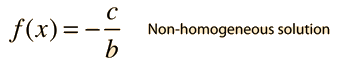It is the nature of differential equations that the sum of solutions is also a solution, so that a general solution can be approached by taking the sum of the two solutions above. The final requirement for the application of the solution to a physical problem is that the solution fits the physical boundary conditions of the problem. The most common situation in physical problems is that the boundary conditions are the values of the function f(x) and its derivatives when x=0. Boundary conditions are often called "initial conditions". For the first order equation, we need to specify one boundary condition. For example:Substituting at x=0 gives: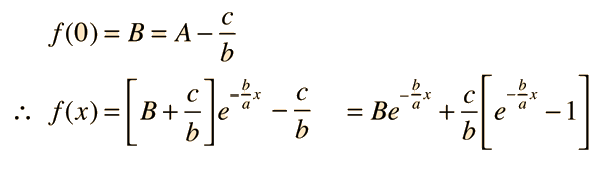Example of capacitor charging
Index

 HyperPhysics****HyperMath*****Differential equations R Nave
Go Back

# Charging a Capacitor

## An application of non-homogeneous differential equations

A first order non-homogeneous differential equationhas a solution of the form :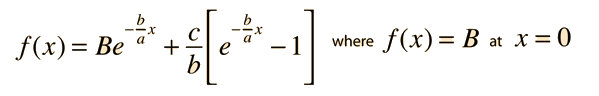.
For the process of charging a capacitor from zero charge with a battery, the equation is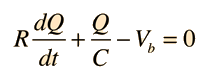.

Using the boundary condition Q=0 at t=0 and identifying the terms corresponding to the general solution, the solutions for the charge on the capacitor and the current are: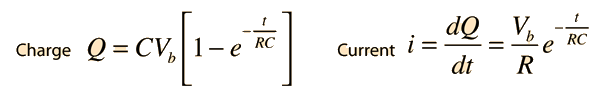.

In this example the constant B in the general solution had the value zero, but if the charge on the capacitor had not been initially zero, the general solution would still give an accurate description of the change of charge with time. The discharge of the capacitor is an example of application of the homogeneous differential equation.

Index

 HyperPhysics****HyperMath*****Differential equations R Nave
Go Back

# Capacitor Discharge

## An application of homogeneous differential equations

A first order homogeneous differential equation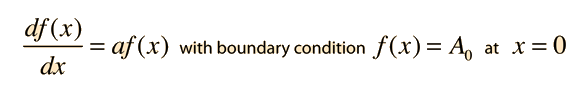has a solution of the form :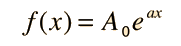.

For the process of discharging a capacitor C, which is initially charged to the voltage of a battery Vb, the equation is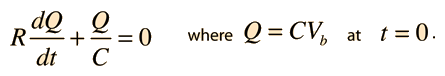Using the boundary condition and identifying the terms corresponding to the general solution, the solutions for the charge on the capacitor and the current are: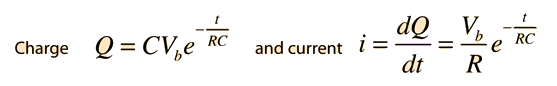.

Since the voltage on the capacitor during the discharge is strictly determined by the charge on the capacitor, it follows the same pattern.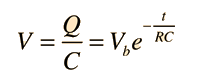.
Index

 HyperPhysics****HyperMath*****Differential equations R Nave
Go Back